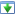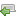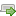Choose a version here. If you've not already started the tutorial, just go with the latest one.

 30 Aug 2014 Preview version Choose 5 Oct 2014 Explicitly adds a specific charset for htmlspecialchars(), and wraps it with a custom function Choose 16 Oct 2014 Updated redirect function to work with vhost subfolders Choose 4 Nov 2014 Minor improvements: added missing docblock, fixed security issue, CSS tweak. Switched hashing method to DEFAULT instead of BCRYPT, this is best practice. Choose ♥ 25 Nov 2014 Improve the notes on getting started, in particular choosing a programmer's editor. Added introduction to mod_rewrite rules. Choose 16 Aug 2018 Some bug fixes, remove compatibility library for earlier version of PHP Choose
OK
NB: There are several versions of this tutorial, each successive one containing additional improvements. If you're in the middle of working through it, please check the versions panel above, to ensure you're not mixing code from different versions.

Excellent, we're now on the home straight! Our functionality is finished, so we'll just do a last round of sprucing up. To start, let's add in a home link for authorised users. This is useful on the restricted pages, since they don't have a home link themselves:`1`
`2`
`3`
` `
` `
`4`
`5`
`6`
`<div class="top-menu">`
` <div class="menu-options">`
` <?php if (isLoggedIn()): ?>`
` <a href="list-posts.php">All posts</a>`
` |`
` <a href="edit-post.php">New post</a>`
`1`
`2`
`3`
`4`
`5`
`6`
`7`
`8`
`<div class="top-menu">`
` <div class="menu-options">`
` <?php if (isLoggedIn()): ?>`
` <a href="index.php">Home</a>`
` |`
` <a href="list-posts.php">All posts</a>`
` |`
` <a href="edit-post.php">New post</a>`

Next, I thought it would be nice to have a comment count for each article on the All Posts page. Add the following changes:`83`
`84`
`85`
`86`
` `
`87`
`88`
`89`
`{`
` \$stmt = \$pdo->query(`
` 'SELECT`
` id, title, created_at, body`
` FROM`
` post`
` ORDER BY`
`83`
`84`
`85`
`86`
`87`
`88`
`89`
`90`
`{`
` \$stmt = \$pdo->query(`
` 'SELECT`
` id, title, created_at, body,`
` (SELECT COUNT(*) FROM comment WHERE comment.post_id = post.id) comment_count`
` FROM`
` post`
` ORDER BY`
`54`
`55`
`56`
` `
` `
` `
`57`
`58`
`59`
` <td>`
` <?php echo convertSqlDate(\$post['created_at']) ?>`
` </td>`
` <td>`
` <a href="edit-post.php?post_id=<?php echo \$post['id']?>">Edit</a>`
` </td>`
`54`
`55`
`56`
`57`
`58`
`59`
`60`
`61`
`62`
` <td>`
` <?php echo convertSqlDate(\$post['created_at']) ?>`
` </td>`
` <td>`
` <?php echo \$post['comment_count'] ?>`
` </td>`
` <td>`
` <a href="edit-post.php?post_id=<?php echo \$post['id']?>">Edit</a>`
` </td>`

Let's take a closer look at how the new query works. The query now looks like this:

``````SELECT
id, title, created_at, body,
(SELECT COUNT(*) FROM comment WHERE comment.post_id = post.id) comment_count
FROM
post
ORDER BY
created_at DESC``````

The new part of this is the bracketed expression on the third line. This sort of SQL is known as a sub-query since it is a query contained inside another one. The result of it can be read like a real column, which we explicitly name by adding `comment_count` at the end of it.

This sub-query will count rows in the `comment` table, based on which post they belong to. Since the job of the outer query is to list posts, we can filter the comment count for each row by making a comparison to the outer table, `post`.

The next change is another easy one: we'll add a header row to the All Posts table:`110`
`111`
`112`
`113`
`114`
` `
`115`
`116`
`117`
` border: 1px solid silver;`
`}`
`#post-list td {`
` padding: 8px;`
`}`
`#post-list tbody tr:nth-child(odd) {`
`110`
`111`
`112`
`113`
`114`
`115`
`116`
`117`
`118`
` border: 1px solid silver;`
`}`
`#post-list td, #post-list th {`
` padding: 8px;`
` text-align: left;`
`}`
`#post-list tbody tr:nth-child(odd) {`
`45`
`46`
`47`
` `
` `
` `
` `
` `
` `
` `
` `
` `
`48`
`49`
`50`
` <form method="post">`
` <table id="post-list">`
` <tbody>`
` <?php foreach (\$posts as \$post): ?>`
` <tr>`
`45`
`46`
`47`
`48`
`49`
`50`
`51`
`52`
`53`
`54`
`55`
`56`
`57`
`58`
`59`
` <form method="post">`
` <table id="post-list">`
` <thead>`
` <tr>`
` <th>Title</th>`
` <th>Creation date</th>`
` <th>Comments</th>`
` <th />`
` <th />`
` </tr>`
` </thead>`
` <tbody>`
` <?php foreach (\$posts as \$post): ?>`
` <tr>`

While we are on the All Posts page, it would make sense to add in a link to the view page for each article. So let's do that now:`58`
`59`
`60`
`61`
` `
` `
`62`
`63`
`64`
` <?php foreach (\$posts as \$post): ?>`
` <tr>`
` <td>`
` <?php echo htmlEscape(\$post['title']) ?>`
` </td>`
` <td>`
` <?php echo convertSqlDate(\$post['created_at']) ?>`
`58`
`59`
`60`
`61`
`62`
`63`
`64`
`65`
`66`
` <?php foreach (\$posts as \$post): ?>`
` <tr>`
` <td>`
` <a`
` href="view-post.php?post_id=<?php echo \$post['id']?>"`
` ><?php echo htmlEscape(\$post['title']) ?></a>`
` </td>`
` <td>`
` <?php echo convertSqlDate(\$post['created_at']) ?>`

Next up is a refactoring that simplifies the code (quite a bit is removed) and reduces the number of calls made to the database. The essence of the modification is in lib/view-post.php — we merge two SQL calls together via another sub-query.

There's quite a few files to modify here, so make sure you get them all.`34`
`35`
`36`
`37`
`38`
`39`
`40`
` <div class="meta">`
` <?php echo convertSqlDate(\$post['created_at']) ?>`
` (<?php echo countCommentsForPost(\$pdo, \$post['id']) ?> comments)`
` </div>`
` <p>`
` <?php echo htmlEscape(\$post['body']) ?>`
`34`
`35`
`36`
`37`
`38`
`39`
`40`
` <div class="meta">`
` <?php echo convertSqlDate(\$post['created_at']) ?>`
` (<?php echo \$post['comment_count'] ?> comments)`
` </div>`
` <p>`
` <?php echo htmlEscape(\$post['body']) ?>`
`125`
`126`
`127`
`128`
`129`
`130`
`131`
`132`
`133`
`134`
`135`
`136`
`137`
`138`
`139`
`140`
`141`
`142`
`143`
`144`
`145`
`146`
`147`
`148`
`149`
`150`
`151`
`152`
`153`
`154`
`155`
` exit();`
`}`
`/**`
` * Returns the number of comments for the specified post`
` *`
` * @param PDO \$pdo`
` * @param integer \$postId`
` * @return integer`
` */`
`function countCommentsForPost(PDO \$pdo, \$postId)`
`{`
` \$sql = "`
` SELECT`
` COUNT(*) c`
` FROM`
` comment`
` WHERE`
` post_id = :post_id`
` ";`
` \$stmt = \$pdo->prepare(\$sql);`
` \$stmt->execute(`
` array('post_id' => \$postId, )`
` );`
` return (int) \$stmt->fetchColumn();`
`}`
`/**`
` * Returns all the comments for the specified post`
` *`
`125`
`126`
`127`
` `
` `
` `
` `
` `
` `
` `
` `
` `
` `
` `
` `
` `
` `
` `
` `
` `
` `
` `
` `
` `
` `
` `
` `
` `
`128`
`129`
`130`
` exit();`
`}`
`/**`
` * Returns all the comments for the specified post`
` *`
`100`
`101`
`102`
`103`
` `
`104`
`105`
`106`
`{`
` \$stmt = \$pdo->prepare(`
` 'SELECT`
` title, created_at, body`
` FROM`
` post`
` WHERE`
`100`
`101`
`102`
`103`
`104`
`105`
`106`
`107`
`{`
` \$stmt = \$pdo->prepare(`
` 'SELECT`
` title, created_at, body,`
` (SELECT COUNT(*) FROM comment WHERE comment.post_id = post.id) comment_count`
` FROM`
` post`
` WHERE`
`2`
`3`
`4`
` `
`5`
`6`
`7`
`10`
`11`
`12`
`13`
`14`
`15`
`16`
`/**`
` * @var \$pdo PDO`
` * @var \$postId integer`
` */`
`?>`
`<form`
` method="post"`
` class="comment-list"`
`>`
` <h3><?php echo countCommentsForPost(\$pdo, \$postId) ?> comments</h3>`
` <?php foreach (getCommentsForPost(\$pdo, \$postId) as \$comment): ?>`
` <div class="comment">`
`2`
`3`
`4`
`5`
`6`
`7`
`8`
`10`
`11`
`12`
`13`
`14`
`15`
`16`
`/**`
` * @var \$pdo PDO`
` * @var \$postId integer`
` * @var \$commentCount integer`
` */`
`?>`
`<form`
` method="post"`
` class="comment-list"`
`>`
` <h3><?php echo \$commentCount ?> comments</h3>`
` <?php foreach (getCommentsForPost(\$pdo, \$postId) as \$comment): ?>`
` <div class="comment">`
`18`
`19`
`20`
` `
`21`
`22`
`23`
`// Connect to the database, run a query, handle errors`
`\$pdo = getPDO();`
`\$row = getPostRow(\$pdo, \$postId);`
`// If the post does not exist, let's deal with that here`
`if (!\$row)`
`18`
`19`
`20`
`21`
`22`
`23`
`24`
`// Connect to the database, run a query, handle errors`
`\$pdo = getPDO();`
`\$row = getPostRow(\$pdo, \$postId);`
`\$commentCount = \$row['comment_count'];`
`// If the post does not exist, let's deal with that here`
`if (!\$row)`

I noticed when doing some testing at this point that posts which have comments cannot be deleted - try that now. You'll get an error known as a foreign key constraint violation; this is what happens when a row is deleted that a foreign key depends on (in our case we are trying to delete a post to which comments are still attached). To fix this, comments should be deleted first, prior to the post being deleted:`3`
`4`
`5`
` `
` `
`6`
`7`
`8`
`12`
`13`
`14`
`15`
`16`
` `
` `
` `
` `
` `
` `
`17`
`18`
`19`
`20`
`21`
`22`
`23`
`24`
`25`
` `
` `
` `
`26`
`27`
`28`
`29`
` `
` `
` `
` `
` `
` `
` `
`30`
`31`
`32`
`/**`
` * Tries to delete the specified post`
` *`
` * @param PDO \$pdo`
` * @param integer \$postId`
` * @return boolean Returns true on successful deletion`
` */`
`function deletePost(PDO \$pdo, \$postId)`
`{`
` \$sql = "`
` DELETE FROM`
` post`
` WHERE`
` id = :id`
` ";`
` \$stmt = \$pdo->prepare(\$sql);`
` if (\$stmt === false)`
` {`
` throw new Exception('There was a problem preparing this query');`
` }`
` \$result = \$stmt->execute(`
` array('id' => \$postId, )`
` );`
` return \$result !== false;`
`}`
`3`
`4`
`5`
`6`
`7`
`8`
`9`
`10`
`12`
`13`
`14`
`15`
`16`
`17`
`18`
`19`
`20`
`21`
`22`
`23`
`24`
`25`
`26`
`27`
`28`
`29`
`30`
`31`
`32`
`33`
`34`
`35`
`36`
`37`
`38`
`39`
`40`
`41`
`42`
`43`
`44`
`45`
`46`
`47`
`48`
`/**`
` * Tries to delete the specified post`
` *`
` * We first delete the comments attached to the post, and then delete the post itself`
` *`
` * @param PDO \$pdo`
` * @param integer \$postId`
` * @return boolean Returns true on successful deletion`
` */`
`function deletePost(PDO \$pdo, \$postId)`
`{`
` \$sqls = array(`
` // Delete comments first, to remove the foreign key objection`
` "DELETE FROM`
` comment`
` WHERE`
` post_id = :id",`
` // Now we can delete the post`
` "DELETE FROM`
` post`
` WHERE`
` id = :id",`
` );`
` foreach (\$sqls as \$sql)`
` {`
` \$stmt = \$pdo->prepare(\$sql);`
` if (\$stmt === false)`
` {`
` throw new Exception('There was a problem preparing this query');`
` }`
` \$result = \$stmt->execute(`
` array('id' => \$postId, )`
` );`
` // Don't continue if something went wrong`
` if (\$result === false)`
` {`
` break;`
` }`
` }`
` return \$result !== false;`
`}`

Whilst we are looking at the database side of things, I noticed that the `user.is_enabled` field hasn't yet been used. This was intended mainly for future enhancements (in particular for a user administration page), but the application can make an initial use of it immediately, by disallowing authenticated features to non-enabled users.

The installer sets the test user to `is_enabled=1` already, so all we need to do is to adjust the SQL statements that fetch data from the user table.`159`
`160`
`161`
` `
`162`
`163`
`164`
`225`
`226`
`227`
` `
`228`
`229`
`230`
` user`
` WHERE`
` username = :username`
` ";`
` \$stmt = \$pdo->prepare(\$sql);`
` \$stmt->execute(`
` user`
` WHERE`
` username = :username`
` ";`
` \$stmt = \$pdo->prepare(\$sql);`
` \$stmt->execute(`
`159`
`160`
`161`
`162`
`163`
`164`
`165`
`225`
`226`
`227`
`228`
`229`
`230`
`231`
` user`
` WHERE`
` username = :username`
` AND is_enabled = 1`
` ";`
` \$stmt = \$pdo->prepare(\$sql);`
` \$stmt->execute(`
` user`
` WHERE`
` username = :username`
` AND is_enabled = 1`
` ";`
` \$stmt = \$pdo->prepare(\$sql);`
` \$stmt->execute(`

Our final improvement is to add some labels and make use of existing form styles:`57`
`58`
`59`
` `
`60`
`61`
`62`
` `
` `
`63`
`64`
` `
`65`
`66`
`67`
`68`
`69`
`70`
`71`
`72`
` `
` `
` `
` `
` `
` `
`73`
`74`
`75`
` <form`
` method="post"`
` >`
` <p>`
` Username:`
` <input`
` type="text"`
` name="username"`
` value="<?php echo htmlEscape(\$username) ?>"`
` />`
` </p>`
` <p>`
` Password:`
` <input type="password" name="password" />`
` </p>`
` <input type="submit" name="submit" value="Login" />`
` </form>`
` </body>`
`57`
`58`
`59`
`60`
`61`
`62`
`63`
`64`
`65`
`66`
`67`
`68`
`69`
`70`
`71`
`72`
`73`
`74`
`75`
`76`
`77`
`78`
`79`
`80`
`81`
`82`
`83`
`84`
`85`
` <form`
` method="post"`
` class="user-form"`
` >`
` <div>`
` <label for="username">`
` Username:`
` </label>`
` <input`
` type="text"`
` id="username"`
` name="username"`
` value="<?php echo htmlEscape(\$username) ?>"`
` />`
` </div>`
` <div>`
` <label for="password">`
` Password:`
` </label>`
` <input`
` type="password"`
` id="password"`
` name="password"`
` />`
` </div>`
` <input type="submit" name="submit" value="Login" />`
` </form>`
` </body>`

Well done, you have finished the tutorial! It is a good idea to regenerate the database one last time, and then give the whole application a thorough testing. Add some posts and comments, both while logged in and while logged out, and delete comments in the admin interface.